# 1. Below are production functions that turn capital (K) and labor (L) into output. For cach of th...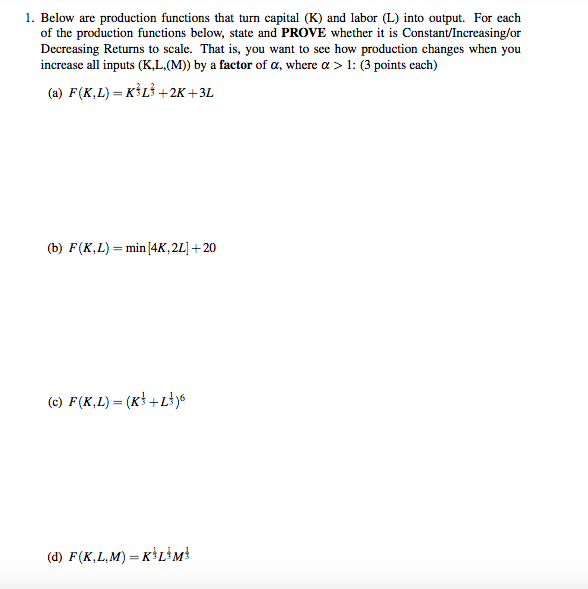1. Below are production functions that turn capital (K) and labor (L) into output. For cach of the production functions below, state and PROVE whether it is Constant/Increasing/or Decreasing Returns to scale. That is, you want to see how production changes when you increase all inputs (K,L, (M)) by a factor of a, where a > 1: (3 points each) (a) F(K,L)-KİLİ+2K +3L (b) F(K, L)=min/4K, 2L1+20 (d) F(K,L,M) KL3M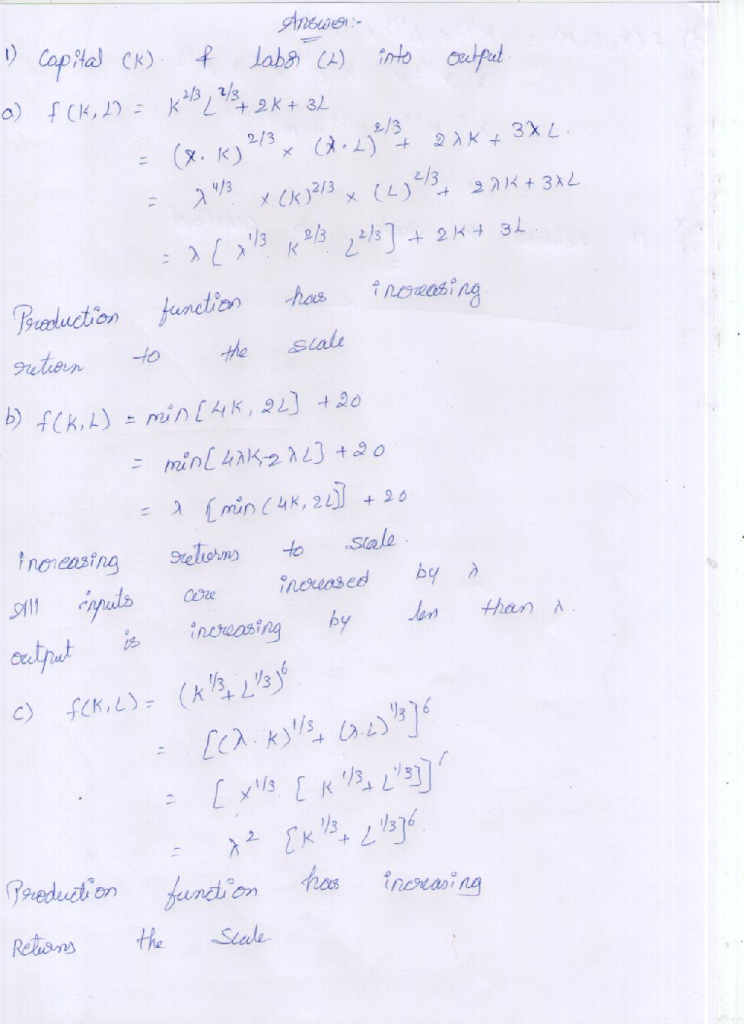#### Earn Coin

Coins can be redeemed for fabulous gifts.

Similar Homework Help Questions
• ### 1. Below are production functions that turn capital (K) and labor (L) into output. For each...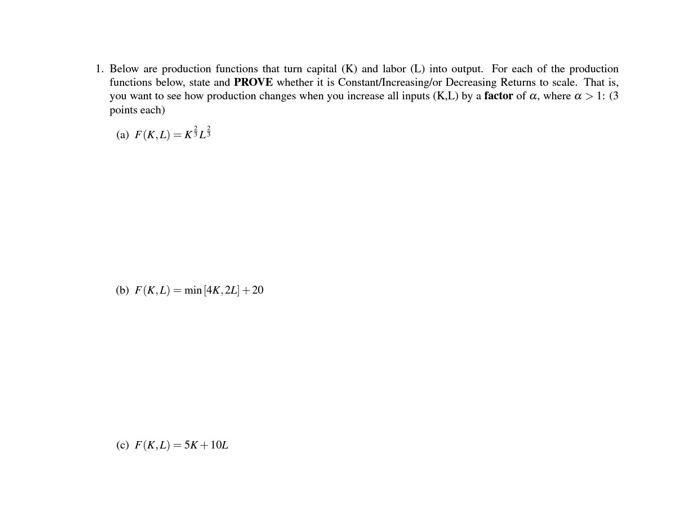1. Below are production functions that turn capital (K) and labor (L) into output. For each of the production functions below, state and PROVE whether it is Constant/Increasing/or Decreasing Returns to scale. That is, you want to see how production changes when you increase all inputs (K.L) by a factor of a, where a > 1: (3 points each) (a) F(KL)=KL (b) F(K,L) = min (4K, 22] + 20 (c) F(K,L) = 5K+10L

• ### 1. Below are production functions that turn capital (K) and labor (L) into output. For each...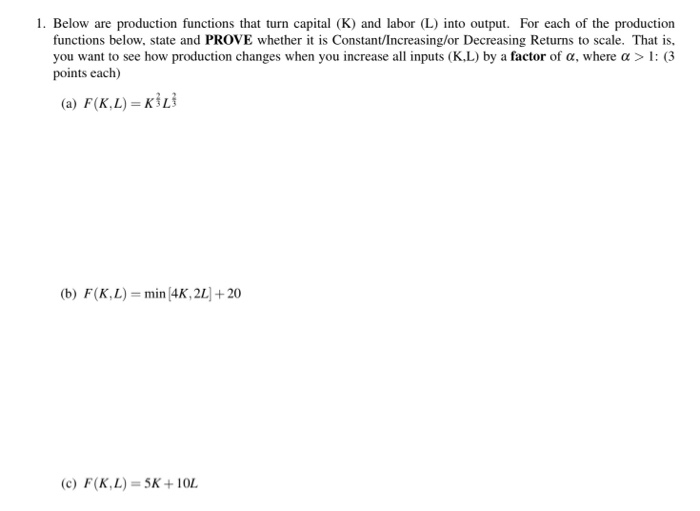1. Below are production functions that turn capital (K) and labor (L) into output. For each of the production functions below, state and PROVE whether it is Constant/Increasing/or Decreasing Returns to scale. That is, you want to see how production changes when you increase all inputs (KL) by a factor of a, where a > 1: (3 points each) (a) F(K.L) = (b) F(KL)= min (4K, 2L + 20 (c) F(K,L) = 5K+ 10L

• ### Below are production functions that turn capital (K) and labor (L) into output. For each of...

Below are production functions that turn capital (K) and labor (L) into output. For each of the production functions below, state and PROVE whether it is Constant/Increasing/or Decreasing Returns to scale. That is, you want to see how production changes when you increase all inputs (K,L,(M)) by a factor of α, where α > 1: (4 points each) a) F(K,L) = K^1/3*L^1/3+2K+3 b) F(K,L) = sqr(K^3+L^3) c) F(K,L) = (K^2/4+L^2/4)^2 d) F(K,L,M) = min(K,L)*M

• ### 4. Below are production functions that turn capital (K) and labor (L) into output. For each...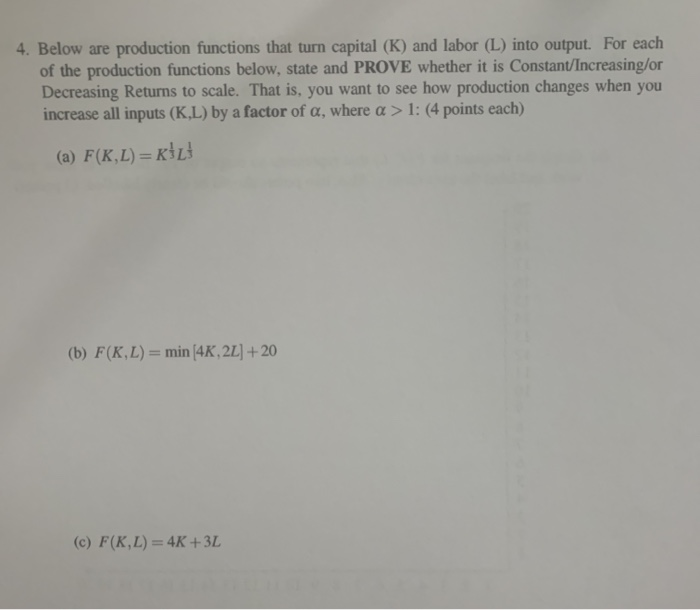4. Below are production functions that turn capital (K) and labor (L) into output. For each of the production functions below, state and PROVE whether it is Constant/Increasing/or Decreasing Returns to scale. That is, you want to see how production changes when you increase all inputs (K.L) by a factor of a, where a > 1: (4 points each) (a) F(K,L) =KİL (b) F(K,L) = min 4K, 2L] + 20 (c) F(K,L) = 4K +3L 5. For this problem you...

• ### 1. Graph the short-run total product curves for each of the following production functions if K...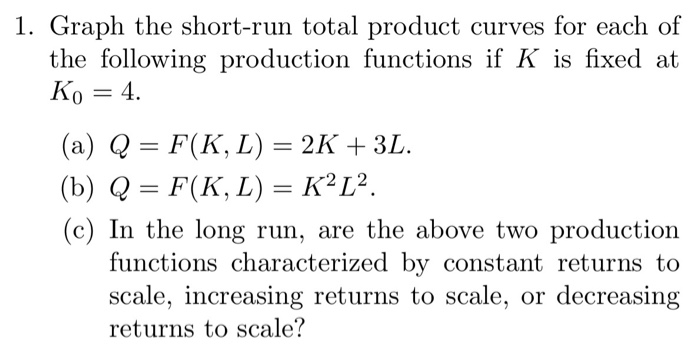1. Graph the short-run total product curves for each of the following production functions if K is fixed at Ko 4 (a) Q = F(K, L) = 2K + 3L. (b) Q = F(K, L) = K2L2. (c) In the long run, are the above two production functions characterized by constant returns to scale, increasing returns to scale, or decreasing returns to scale?

• ### 1. A production function is given by f(K, L) = L/2+ v K. Given this form,...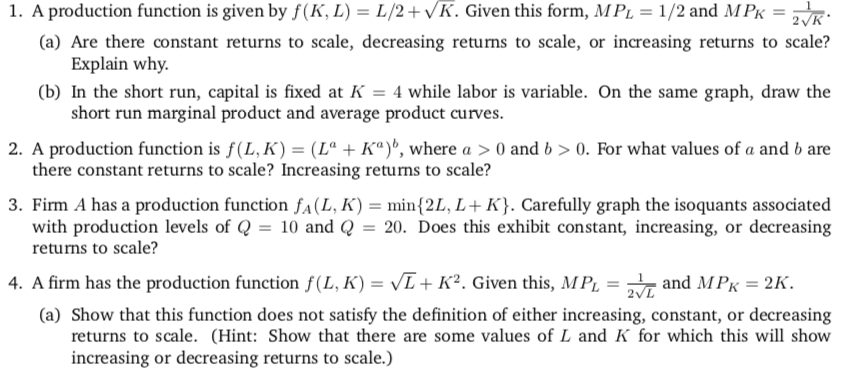1. A production function is given by f(K, L) = L/2+ v K. Given this form, MPL = 1/2 and MPK-2 K (a) Are there constant returns to scale, decreasing returns to scale, or increasing returns to scale? (b) In the short run, capital is fixed at -4 while labor is variable. On the same graph, draw the 2. A production function is f(LK)-(L" + Ka)", where a > 0 and b > 0, For what values of a and...

• ### 1. For the following production functions, find the marginal rate of technical substitu- tion (MRTSLK). Does the produc...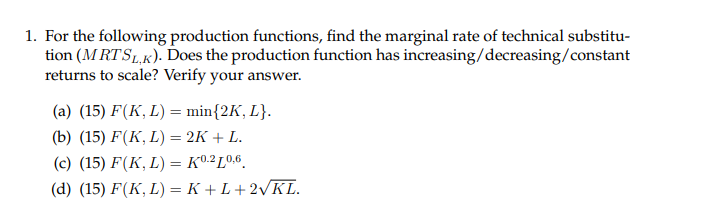1. For the following production functions, find the marginal rate of technical substitu- tion (MRTSLK). Does the production function has increasing/decreasing/constant returns to scale? Verify your answer (a) (15) F(K, L) = min{2K, L}. (b) (15) F(К, L) — 2K + L. (c) (15) F(K, L) = K0.2L0,6. (d) (15) F(K, L) — К +L+2VKL.

• ### 5. Determine whether each of the following production functions displays constant, increasing, or decreasing returns to...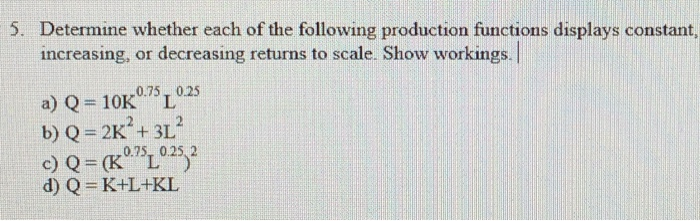5. Determine whether each of the following production functions displays constant, increasing, or decreasing returns to scale. Show workings. a) Q= 10K 0.75, 0.25 b) Q = 2K+ + 3L c) Q = (Kº75 0.25 2 d) Q=K+L+KL

• ### Does the production function of table 7-1 below show constant, increasing, or decreasing return to scale...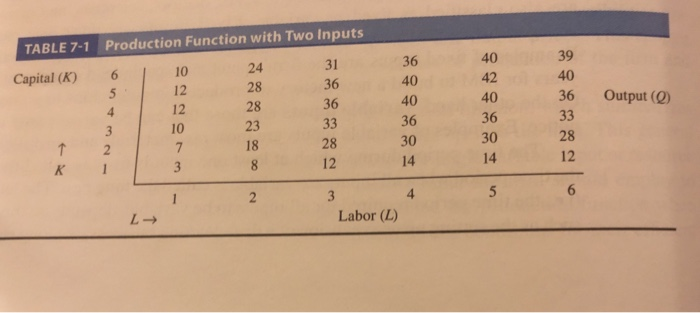Does the production function of table 7-1 below show constant, increasing, or decreasing return to scale if the firm increases the quantity of labor and capital used from (a) 2L and 2K to 4L and 4K? (b) 2L and 4K to 3L and 6K? TABLE 7-1 Production Function with Two Inputs 36 40 40 36 30 14 40 42 40 36 30 14 39 40 36 Output (0) 10 12 12 10 24 28 28 23 18 31 36 36...

• ### Suppose that a companies production function is given by: f(L;K) = (10K^3L^2)/(L+K) a) Does this production...

Suppose that a companies production function is given by: f(L;K) = (10K^3L^2)/(L+K) a) Does this production function exhibit increasing, constant, or decreasing returns to scale? Algebraically justify your answer. b) If there is a wage of 10 and a rental rate of capital of 1, then find the company's expansion path.